## Based on the above information answer the following:

This question is inspired from Example 37 - Chapter 6 Class 12 (AOD) - Maths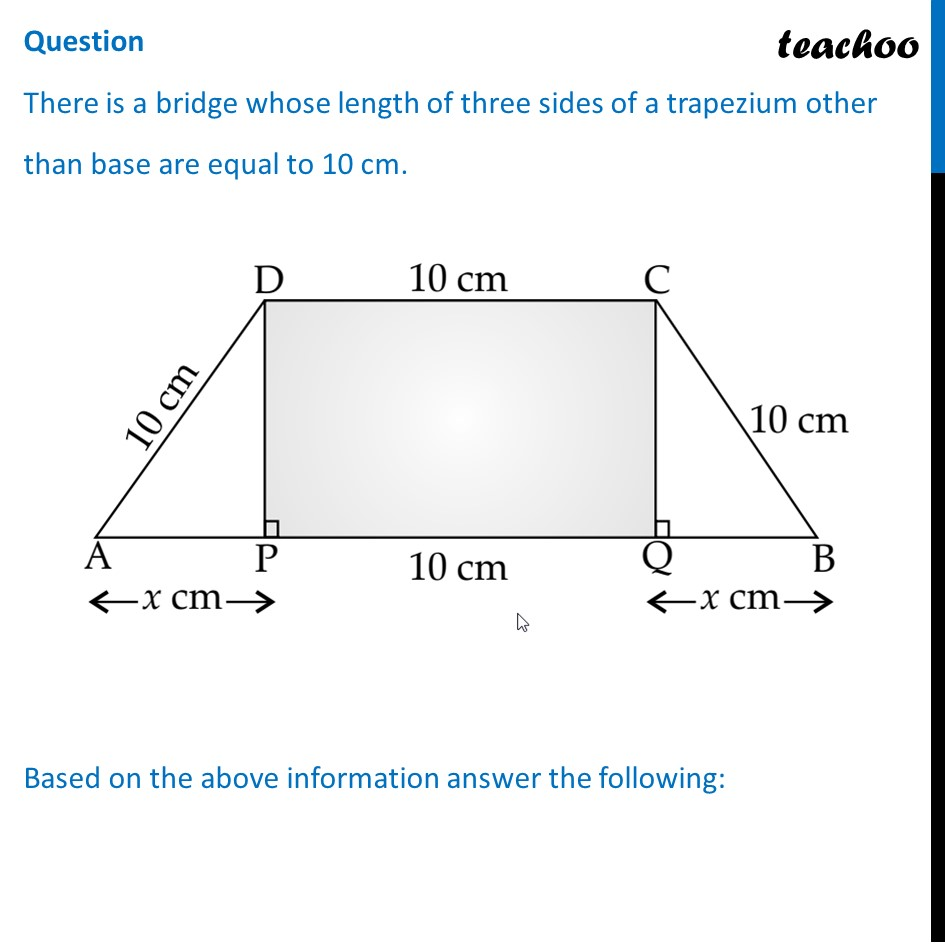## (D) x 2 − 100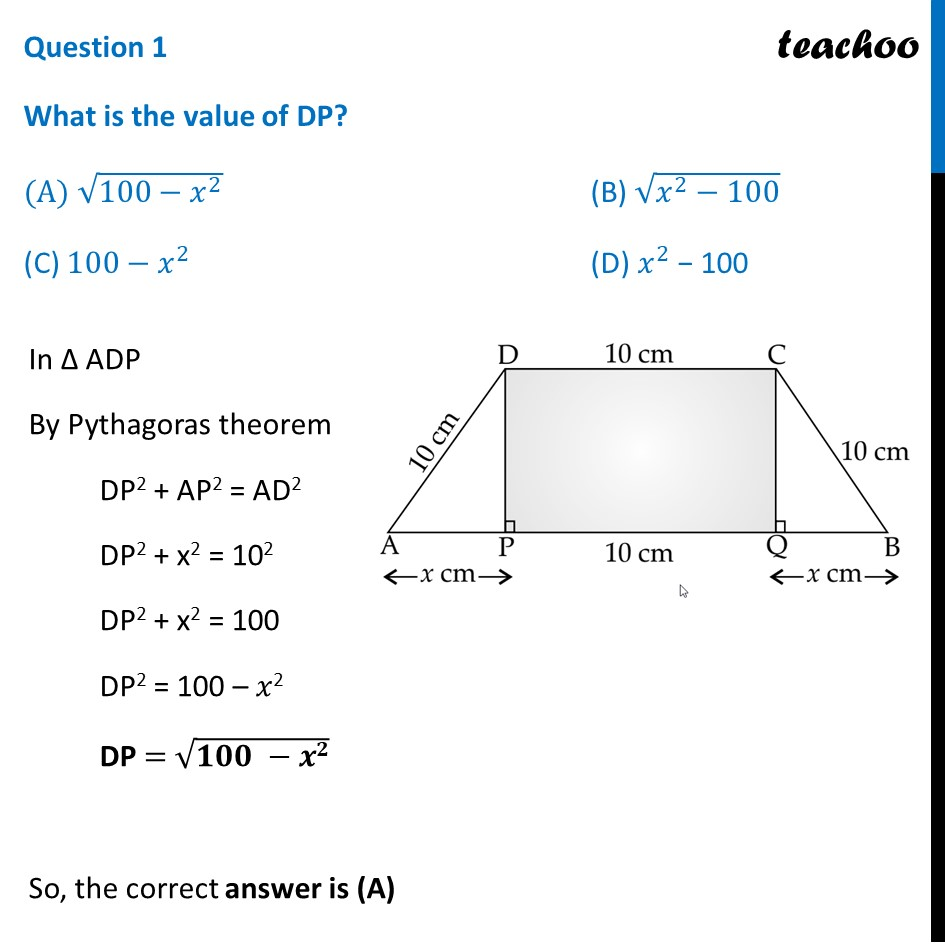## (D) ( x + 10)(100 - x 2 )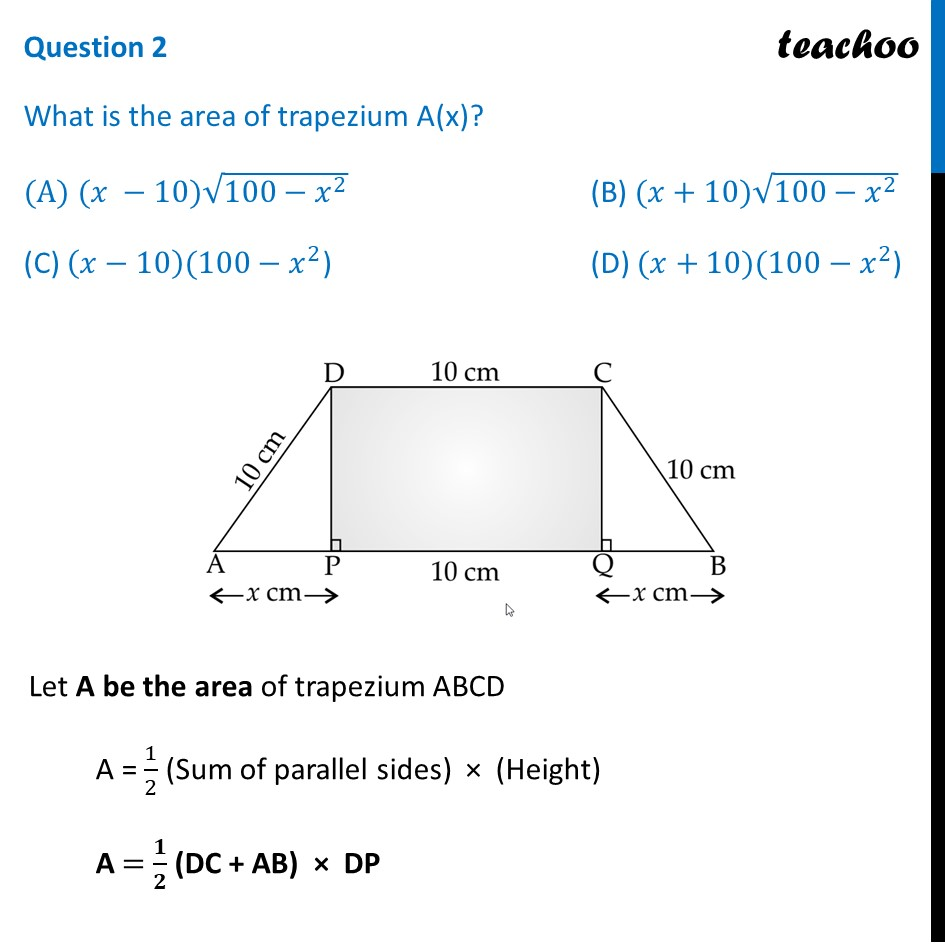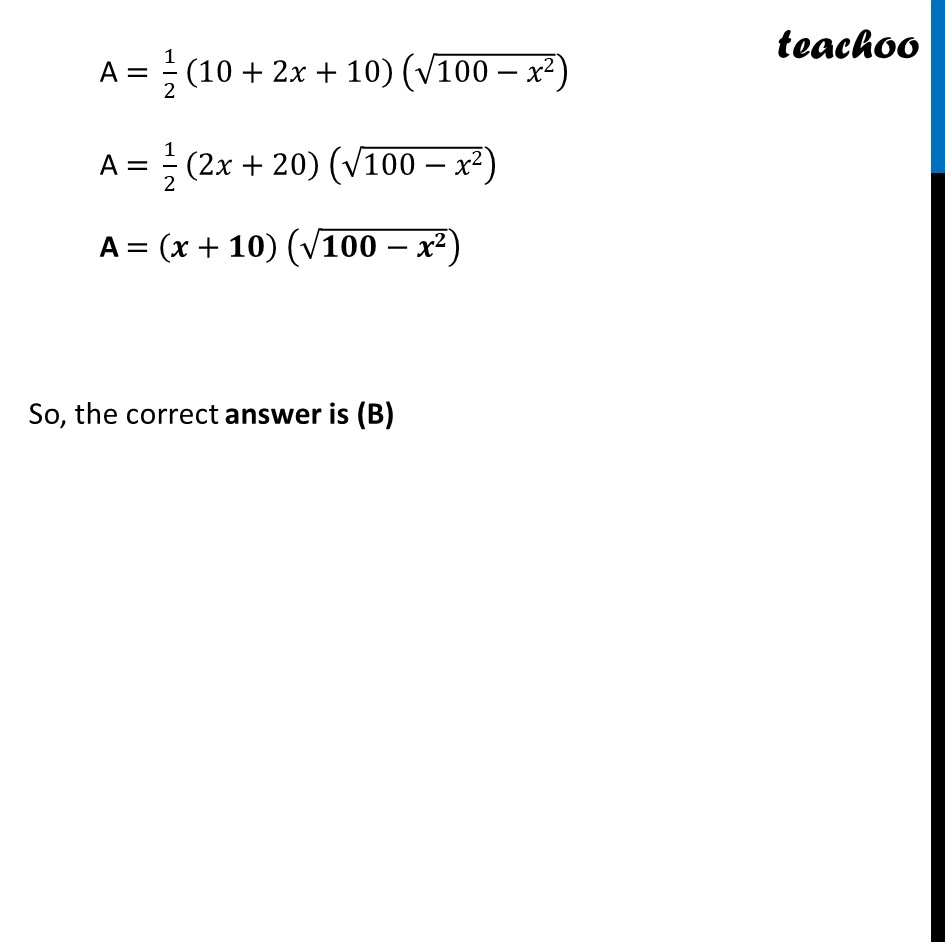## (D) 5,10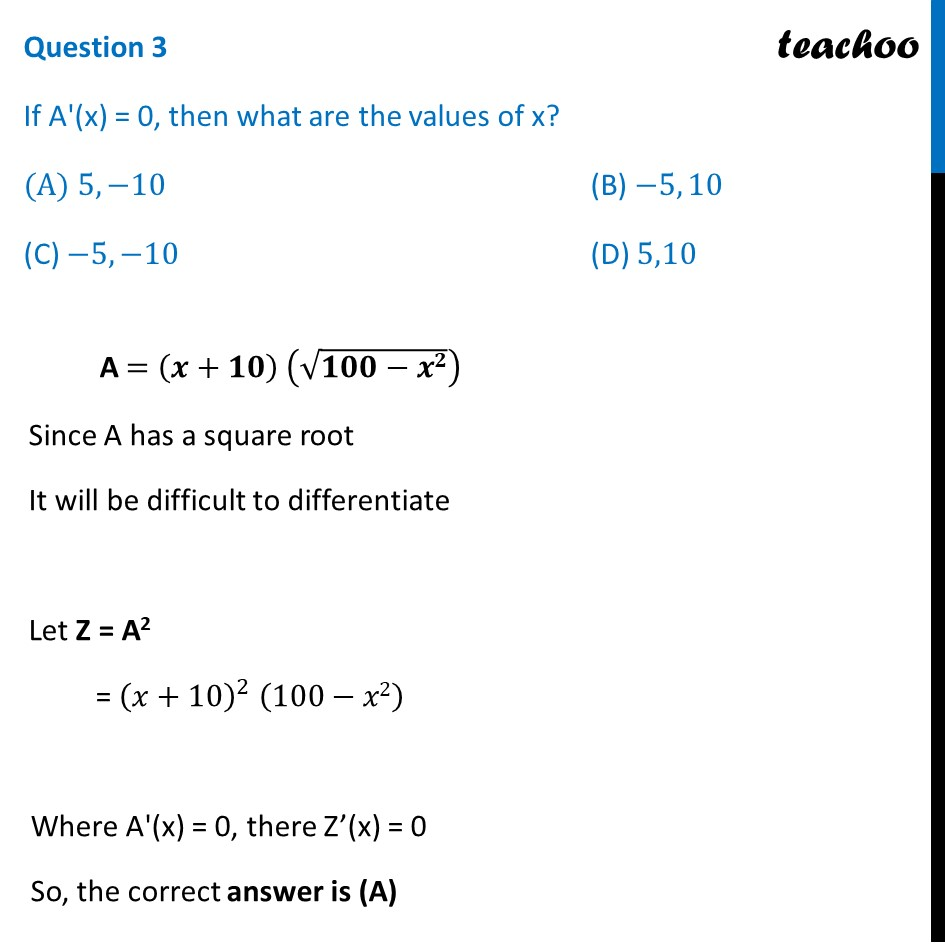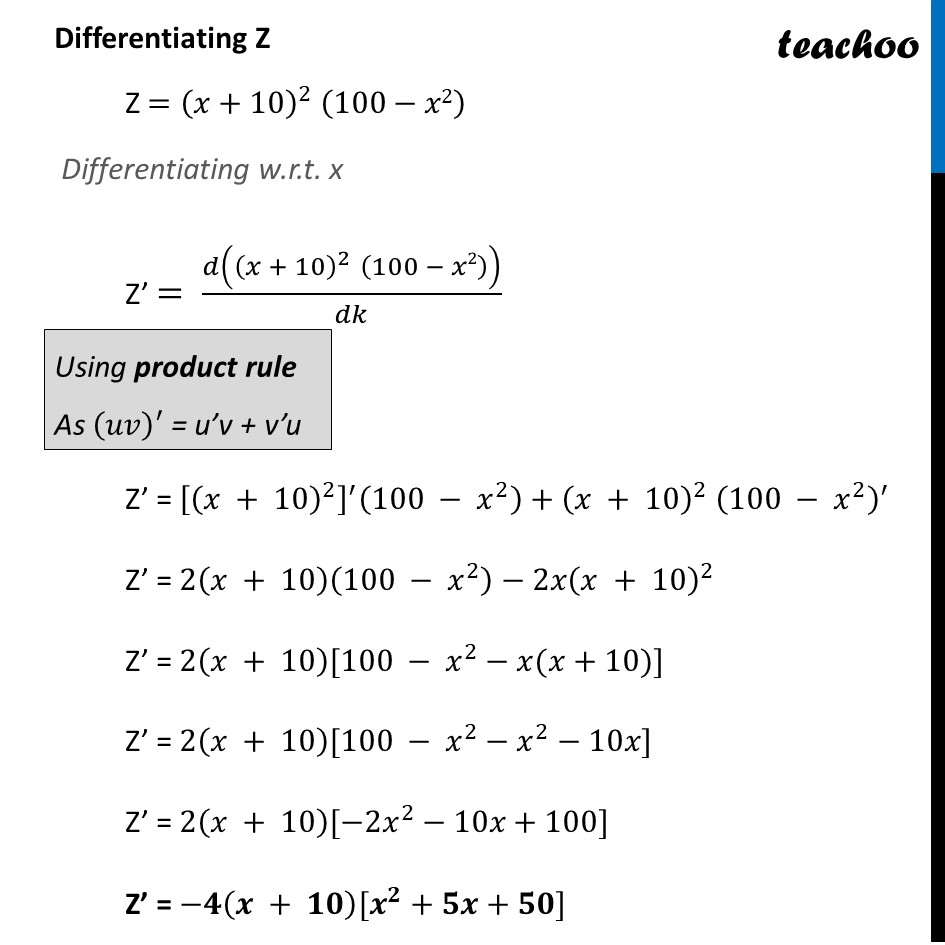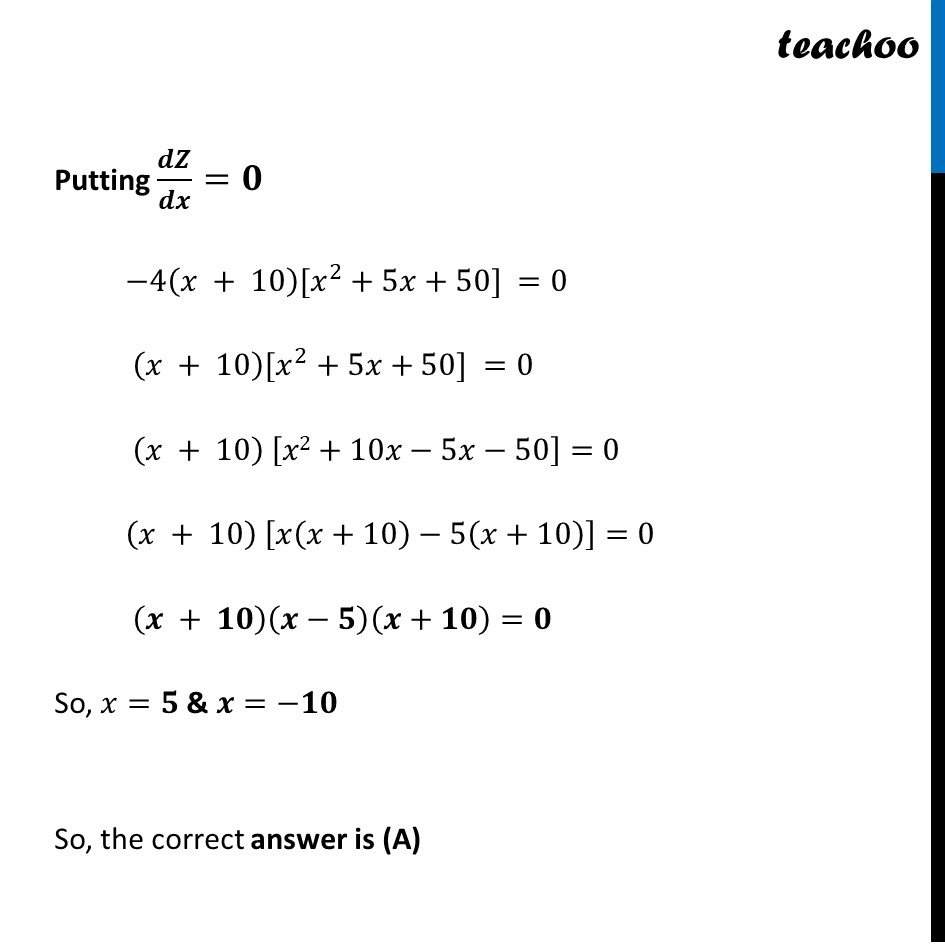## (D) 75 √7  cm 2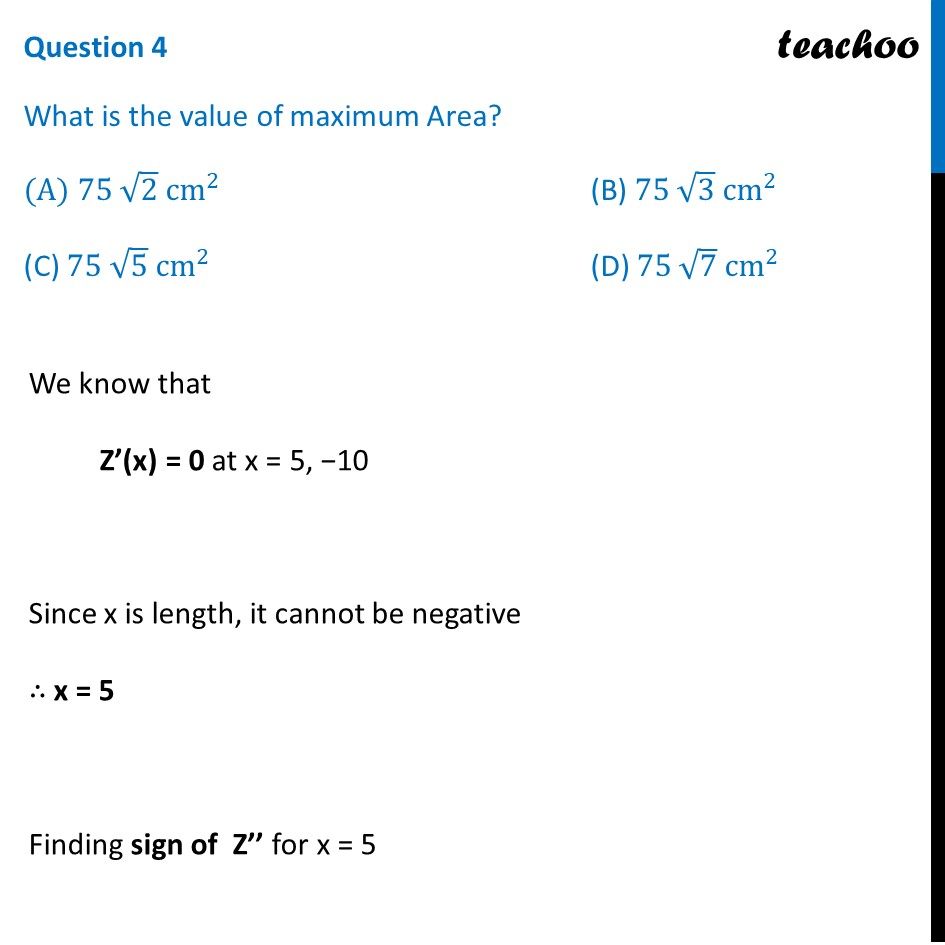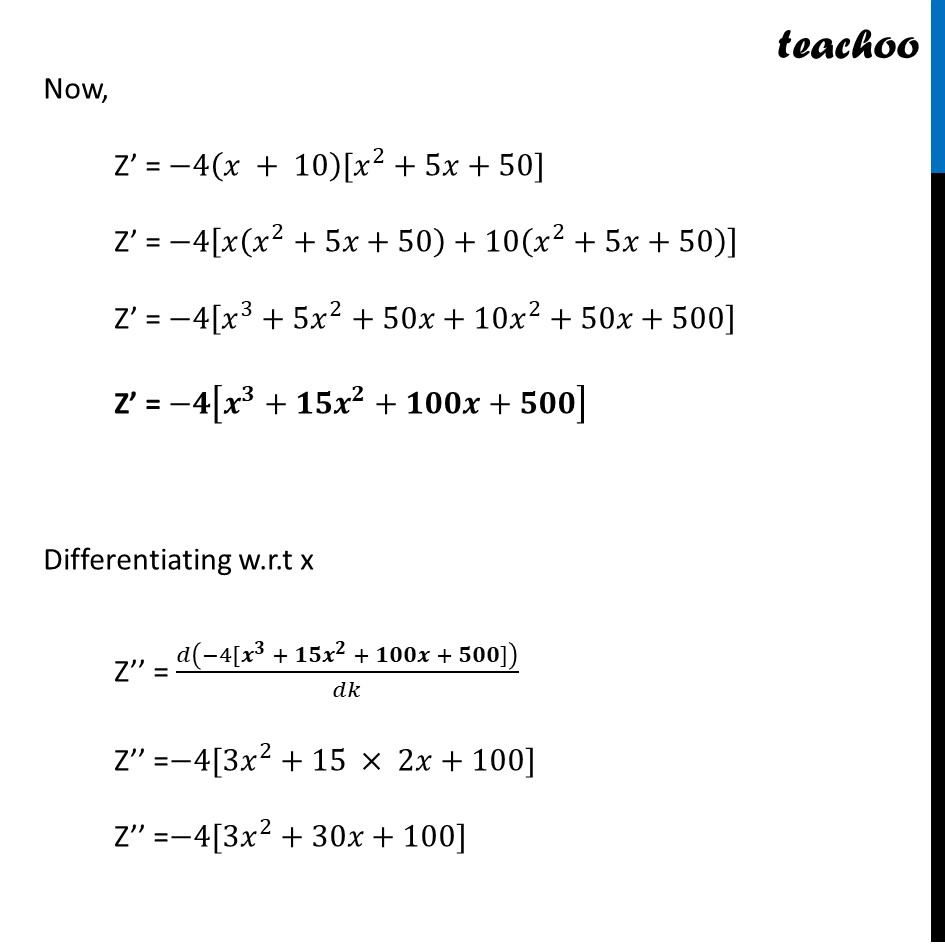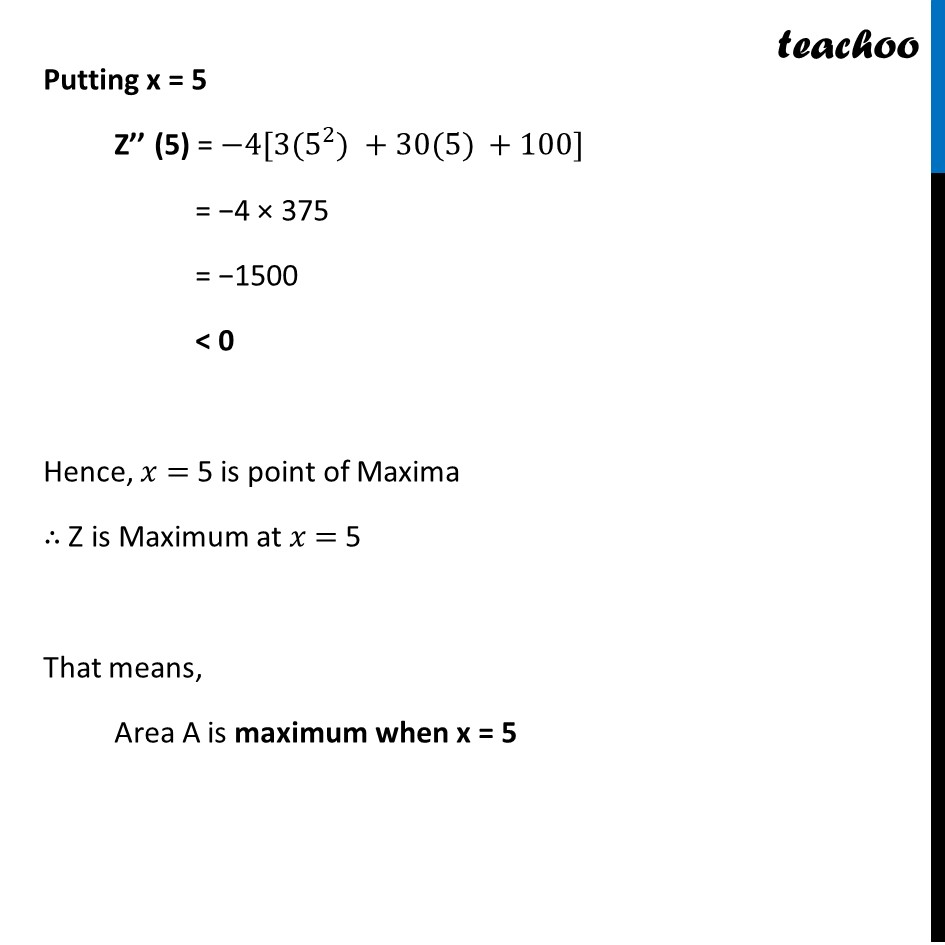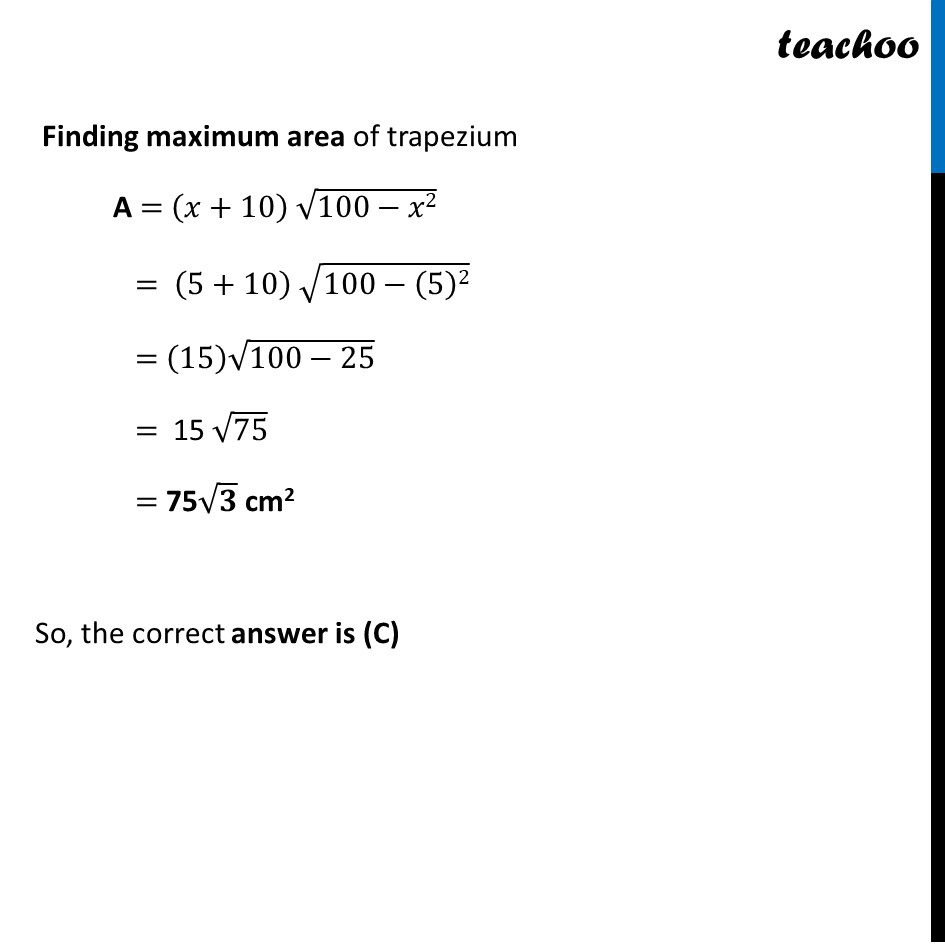1. Chapter 6 Class 12 Application of Derivatives (Term 1)
2. Serial order wise
3. Case Based Questions (MCQ)

Transcript

Question There is a bridge whose length of three sides of a trapezium other than base are equal to 10 cm. Based on the above information answer the following: Question 1 What is the value of DP? (A) √(100−𝑥^2 ) (B) √(𝑥^2−100) (C) 100−𝑥^2 (D)〖 𝑥〗^2 − 100 In Δ ADP By Pythagoras theorem DP2 + AP2 = AD2 DP2 + x2 = 102 DP2 + x2 = 100 DP2 = 100 – 𝑥2 DP = √(𝟏𝟎𝟎 −𝒙𝟐) So, the correct answer is (A) Question 2 What is the area of trapezium A(x)? (A) (𝑥 −10)√(100−𝑥^2 ) (B) (𝑥+10)√(100−𝑥^2 ) (C) (𝑥−10)(100−𝑥^2) (D) (𝑥+10)(100−𝑥^2 ") " Let A be the area of trapezium ABCD A = 1/2 (Sum of parallel sides) × (Height) A = 𝟏/𝟐 (DC + AB) × DP A = 1/2 (10+2𝑥+10) (√(100−𝑥2)) A = 1/2 (2𝑥+20) (√(100−𝑥2)) A = (𝒙+𝟏𝟎) (√(𝟏𝟎𝟎−𝒙𝟐)) So, the correct answer is (B) Question 3 If A'(x) = 0, then what are the values of x? (A) 5,−10 (B) −5, 10 (C) −5,−10 (D) 5,10 A = (𝒙+𝟏𝟎) (√(𝟏𝟎𝟎−𝒙𝟐)) Since A has a square root It will be difficult to differentiate Let Z = A2 = (𝑥+10)^2 (100−𝑥2) Where A'(x) = 0, there Z’(x) = 0 So, the correct answer is (A) Differentiating Z Z =(𝑥+10)^2 " " (100−𝑥2) Differentiating w.r.t. x Z’ = 𝑑((𝑥 + 10)^2 " " (100 − 𝑥2))/𝑑𝑘 Using product rule As (𝑢𝑣)′ = u’v + v’u Z’ = [(𝑥 + 10)^2 ]^′ (100 − 𝑥^2 )+(𝑥 + 10)^2 " " (100 − 𝑥^2 )^′ Z’ = 2(𝑥 + 10)(100 − 𝑥^2 )−2𝑥(𝑥 + 10)^2 Z’ = 2(𝑥 + 10)[100 − 𝑥^2−𝑥(𝑥+10)] Z’ = 2(𝑥 + 10)[100 − 𝑥^2−𝑥^2−10𝑥] Z’ = 2(𝑥 + 10)[−2𝑥^2−10𝑥+100] Z’ = −𝟒(𝒙 + 𝟏𝟎)[𝒙^𝟐+𝟓𝒙+𝟓𝟎] Putting 𝒅𝒁/𝒅𝒙=𝟎 −4(𝑥 + 10)[𝑥^2+5𝑥+50] =0 (𝑥 + 10)[𝑥^2+5𝑥+50] =0 (𝑥 + 10) [𝑥2+10𝑥−5𝑥−50]=0 (𝑥 + 10) [𝑥(𝑥+10)−5(𝑥+10)]=0 (𝒙 + 𝟏𝟎)(𝒙−𝟓)(𝒙+𝟏𝟎)=𝟎 So, 𝑥=𝟓 & 𝒙=−𝟏𝟎 So, the correct answer is (A) Question 4 What is the value of maximum Area? (A) 75 √2 cm^2 (B) 75 √3 cm^2 (C) 75 √5 cm^2 (D) 75 √7 cm^2 We know that Z’(x) = 0 at x = 5, −10 Since x is length, it cannot be negative ∴ x = 5 Finding sign of Z’’ for x = 5 Now, Z’ = −4(𝑥 + 10)[𝑥^2+5𝑥+50] Z’ = −4[𝑥(𝑥^2+5𝑥+50)+10(𝑥^2+5𝑥+50)] Z’ = −4[𝑥^3+5𝑥^2+50𝑥+10𝑥^2+50𝑥+500] Z’ = −𝟒[𝒙^𝟑+𝟏𝟓𝒙^𝟐+𝟏𝟎𝟎𝒙+𝟓𝟎𝟎] Differentiating w.r.t x Z’’ = 𝑑(−4[𝒙^𝟑 + 𝟏𝟓𝒙^𝟐 + 𝟏𝟎𝟎𝒙 + 𝟓𝟎𝟎])/𝑑𝑘 Z’’ =−4[3𝑥^2+15 × 2𝑥+100] Z’’ =−4[3𝑥^2+30𝑥+100] Putting x = 5 Z’’ (5) = −4[3(5^2) +30(5) +100] = −4 × 375 = −1500 < 0 Hence, 𝑥 = 5 is point of Maxima ∴ Z is Maximum at 𝑥 = 5 That means, Area A is maximum when x = 5 Finding maximum area of trapezium A = (𝑥+10) √(100−𝑥2) = (5+10) √(100−(5)2) = (15) √(100−25) = 15 √75 = 75√𝟑 cm2 So, the correct answer is (C)